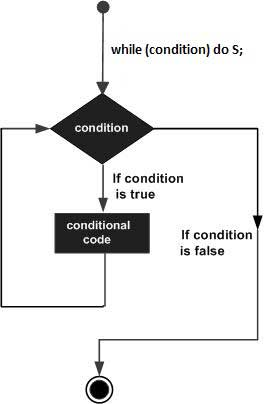## Pascal - While-do Loops

Contents[Hide]

A while-do loop statement in Pascal allows repetitive computations till some test condition is satisfied. In other words it repeatedly executes a target statement as long as a given condition is true.

## 1. Syntax:

The syntax of a while-do loop is:

```while (condition) do S;
```

Where condition is a Boolean or relational expression whose value would be true or false and S is a simple statement or group of statements within BEGIN ... END block.

For example,

```while number > 0 do
begin
sum := sum + number;
number := number -2;
end;
```

When the condition becomes false, program control passes to the line immediately following the loop.

## 2. Flow Diagram:Here key point of the while loop is that the loop might not ever run. When the condition is tested and the result is false, the loop body will be skipped and the first statement after the while loop will be executed.

## 3. Example:

```program whileLoop;
var
a: integer;
begin
a :=10;
while  a 20  do
begin
writeln('value of a: ', a);
a := a +1;
end;
end.
```

When the above code is compiled and executed, it produces following result:

```value of a: 10
value of a: 11
value of a: 12
value of a: 13
value of a: 14
value of a: 15
value of a: 16
value of a: 17
value of a: 18
value of a: 19

```